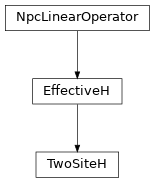# TwoSiteH¶

Inheritance DiagramMethods

 TwoSiteH.__init__(env, i0[, combine, move_right]) Return the hermitian conjugate of self. TwoSiteH.combine_Heff(env[, left, right]) Combine LP and RP with W to form LHeff and RHeff. Combine the legs of theta, such that it fits to how we combined the legs of self. TwoSiteH.matvec(theta) Apply the effective Hamiltonian to theta. Contract self to a matrix. TwoSiteH.update_LP(env, i[, U]) Equivalent to env.get_LP(i, store=True); optimized for combine. TwoSiteH.update_RP(env, i[, VH]) Equivalent to env.get_RP(i, store=True); optimized for combine.

Class Attributes and Properties

class tenpy.algorithms.mps_common.TwoSiteH(env, i0, combine=False, move_right=True)[source]

Bases: EffectiveH

Class defining the two-site effective Hamiltonian for Lanczos.

The effective two-site Hamiltonian looks like this:

|        .---       ---.
|        |    |   |    |
|       LP----W0--W1---RP
|        |    |   |    |
|        .---       ---.


If combine is True, we define LHeff and RHeff, which are the contractions of LP with W0, and RP with W1, respectively.

Parameters
• env (MPOEnvironment) – Environment for contraction <psi|H|psi>.

• i0 (int) – Left-most site of the MPS it acts on.

• combine (bool) – Whether to combine legs into pipes. This combines the virtual and physical leg for the left site (when moving right) or right side (when moving left) into pipes. This reduces the overhead of calculating charge combinations in the contractions, but one matvec() is formally more expensive, $$O(2 d^3 \chi^3 D)$$.

• move_right (bool) – Whether the the sweep is moving right or left for the next update. Ignored for the TwoSiteH.

i0

Left-most site of the MPS it acts on.

Type

int

combine

Whether to combine legs into pipes. This combines the virtual and physical leg for the left site and right site into pipes. This reduces the overhead of calculating charge combinations in the contractions, but one matvec() is formally more expensive, $$O(2 d^3 \chi^3 D)$$.

Type

bool

length

Number of (MPS) sites the effective hamiltonian covers.

Type

int

acts_on

Labels of the state on which self acts. NB: class attribute. Overwritten by normal attribute, if combine.

Type

list of str

LHeff

Left part of the effective Hamiltonian. Labels '(vR*.p0)', 'wR', '(vR.p0*)' for bra, MPO, ket.

Type

Array

RHeff

Right part of the effective Hamiltonian. Labels '(p1*.vL)', 'wL', '(p1.vL*)' for ket, MPO, bra.

Type

Array

LP, W0, W1, RP

Tensors making up the network of self.

Type

Array

matvec(theta)[source]

Apply the effective Hamiltonian to theta.

Parameters

theta (Array) – Labels: vL, p0, p1, vR if combine=False, (vL.p0), (p1.vR) if True

Returns

Product of theta and the effective Hamiltonian.

Return type

theta Array

combine_Heff(env, left=True, right=True)[source]

Combine LP and RP with W to form LHeff and RHeff.

Combine LP with W0 and RP with W1 to get the effective parts of the Hamiltonian with piped legs.

Parameters
• env (MPOEnvironment) – Environment for contraction <psi|H|psi>.

• left (bool) – The mixer might need only one of LHeff/RHeff after the Lanczos optimization even for combine=True. These flags allow to calculate them specifically.

• right (bool) – The mixer might need only one of LHeff/RHeff after the Lanczos optimization even for combine=True. These flags allow to calculate them specifically.

combine_theta(theta)[source]

Combine the legs of theta, such that it fits to how we combined the legs of self.

Parameters

theta (Array) – Wave function with labels 'vL', 'p0', 'p1', 'vR'

Returns

theta – Wave function with labels 'vL', 'p0', 'p1', 'vR'

Return type

Array

to_matrix()[source]

Contract self to a matrix.

Equivalent to env.get_LP(i, store=True); optimized for combine.
Equivalent to env.get_RP(i, store=True); optimized for combine.